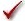## Please enable JavaScript to view this site.

 Navigation: PRINCIPLES OF STATISTICS > Analysis checklists Analysis checklist: Repeated-measures one-way ANOVA

Repeated measures one-way ANOVA compares the means of three or more matched groups. Read elsewhere to learn about choosing a test, and interpreting the results.

###Was the matching effective?

The whole point of using a repeated-measures test is to control for experimental variability. Some factors you don't control in the experiment will affect all the measurements from one subject equally, so will not affect the difference between the measurements in that subject. By analyzing only the differences, therefore, a matched test controls for some of the sources of scatter.

The matching should be part of the experimental design and not something you do after collecting data. Prism tests the effectiveness of matching with an F test (distinct from the main F test of differences between columns). If the P value for matching is large (say larger than 0.05), you should question whether it made sense to use a repeated-measures test. Ideally, your choice of whether to use a repeated-measures test should be based not only on this one P value, but also on the experimental design and the results you have seen in other similar experiments.

###Are the subjects independent?

The results of repeated-measures ANOVA only make sense when the subjects are independent. Prism cannot test this assumption. You must think about the experimental design. For example, the errors are not independent if you have six rows of data, but these were obtained from three animals, with duplicate measurements in each animal. In this case, some factor may affect the measurements from one animal. Since this factor would affect data in two (but not all) rows, the rows (subjects) are not independent.

###Is the random variability distributed according to a Gaussian distribution?

Repeated-measures ANOVA assumes that each measurement is the sum of an overall mean, a treatment effect (the average difference between subjects given a particular treatment and the overall mean), an individual effect (the average difference between measurements made in a certain subject and the overall mean) and a random component. Furthermore, it assumes that the random component follows a Gaussian distribution and that the standard deviation does not vary between individuals (rows) or treatments (columns). While this assumption is not too important with large samples, it can be important with small sample sizes. Prism does not test for violations of this assumption.

###Is there only one factor?

One-way ANOVA compares three or more groups defined by one factor. For example, you might compare a control group, with a drug treatment group and a group treated with drug plus antagonist. Or you might compare a control group with five different drug treatments.

Some experiments involve more than one factor. For example, you might compare three different drugs in men and women. There are two factors in that experiment: drug treatment and gender. Similarly, there are two factors if you wish to compare the effect of drug treatment at several time points. These data need to be analyzed by two-way ANOVA, also called two-factor ANOVA.

###Is the factor “fixed” rather than “random”?

Prism performs Type I ANOVA, also known as fixed-effect ANOVA. This tests for differences among the means of the particular groups you have collected data from. Type II ANOVA, also known as random-effect ANOVA, assumes that you have randomly selected groups from an infinite (or at least large) number of possible groups, and that you want to reach conclusions about differences among ALL the groups, even the ones you didn't include in this experiment. Type II random-effects ANOVA is rarely used, and Prism does not perform it.

WIth repeated measures, Prism can fit a mixed effects model. This model assumes the differences among subjects (or litters...) is random. But it assumes the factor that defines which column each value is entered into is fixed.

###Can you accept the assumption of circularity or sphericity?

Repeated-measures ANOVA assumes that the random error truly is random. A random factor that causes a measurement in one subject to be a bit high (or low) should have no affect on the next measurement in the same subject. This assumption is called circularity or sphericity. It is closely related to another term you may encounter, compound symmetry.

Repeated-measures ANOVA is quite sensitive to violations of the assumption of circularity. If the assumption is violated, the P value will be too low. One way to violate this assumption is to make the repeated measurements in too short a time interval, so that random factors that cause a particular value to be high (or low) don't wash away or dissipate before the next measurement. To avoid violating the assumption, wait long enough between treatments so the subject is essentially the same as before the treatment. When possible, also randomize the order of treatments.

You only have to worry about the assumption of circularity when you perform a repeated-measures experiment, where each row of data represents repeated measurements from a single subject. It is impossible to violate the assumption with randomized block experiments, where each row of data represents data from a matched set of subjects.

If you cannot accept the assumption of sphericity, you can specify that on the Parameters dialog. In that case, Prism will take into account possible violations of the assumption (using the method of Geisser and Greenhouse) and report a higher P value.

###If any values are missing, was that due to a random event?

Starting with Prism 8, repeated measures data can be calculated with missing values by fitting a mixed model. But the results can only be interpreted if the reason for the value being missing is random. If a value is missing because it was too high to measure (or too low), then it is not missing randomly. If values are missing because a treatment is toxic, then the values are not randomly missing.